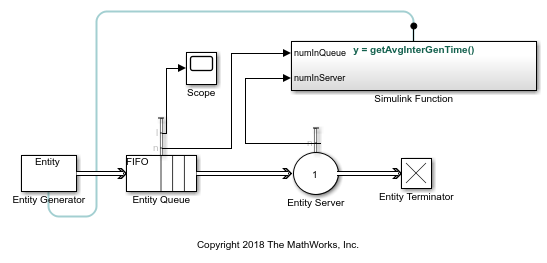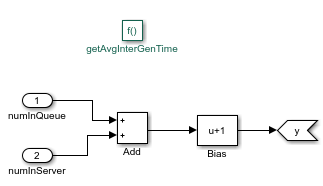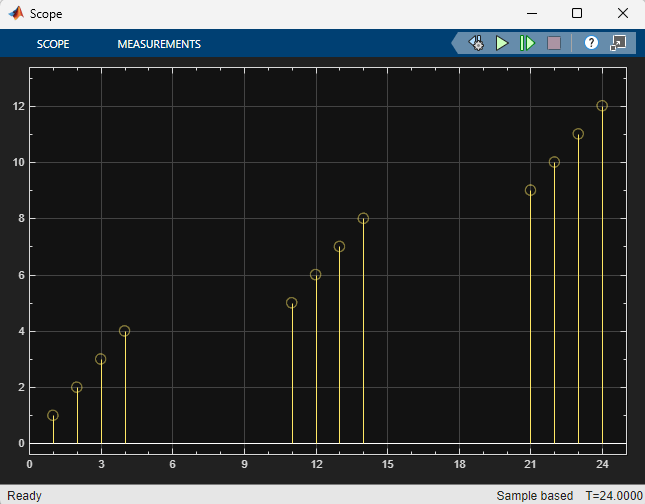Main Content

# Adjust Entity Generation Times Through Feedback

This example shows a queuing system in which feedback influences the arrival rate. The goal of the feedback loop is to stabilize the entity queue by slowing the entity generation rate of the Entity Generator block as more entities accumulate in the Entity Queue block and the Entity Server block.

The diagram shows a simple queuing system with an Entity Generator, an Entity Queue, an Entity Server, and an Entity Terminator block. For more information about building this simple queuing system, see Create a Discrete-Event Model.The capacity of the Entity Server block is `1`. This causes an increase in the queue length without feedback. The goal is to regulate entity intergeneration time based on the size of the queue and the number of entities waiting to be served.

• In the Entity Generator block, select `MATLAB action` as the Time source. Add this code to the Intergeneration time action field.

```persistent rngInit; ```
```if isempty(rngInit) seed = 12345; rng(seed); rngInit = true; end ```
```% Pattern: Exponential distribution mu = getAvgInterGenTime(); dt = -mu*log(1-rand()); ```

The entity intergeneration time `dt` is generated from an exponential distribution with mean `mu`, which is determined by the function `getAvgInterGenTime()`.

• In the Entity Queue block, in the Statistics tab, select the Number of entities in block, n and Average queue length, l as output statistics.

• In the Entity Server block, select `MATLAB action` as the Service time source. Add this code to the Service time action field.

```persistent rngInit; if isempty(rngInit) seed = 67868; rng(seed); rngInit = true; end ```
```% Pattern: Exponential distribution mu = 3; dt = -mu*log(1-rand()); ```
```The service time |dt| is drawn from an exponential distribution with mean |3|.```
• In the Entity Server block, in the Statistics tab, select the Number of entities in block, n as output statistics.

• Add a Simulink Function block. On the Simulink Function block, double-click the function signature and enter `y = getAvgInterGenTime()`.

• In the Simulink Function block:1. Add two In1 blocks and rename them as numInQueue and numInServer.

2. numInQueue represents the current number of entities accumulated in the queue and numInServer represents the current number of entities accumulated in the server.

3. Use Add block to add these two inputs.

4. Use a Bias block and set the `Bias` parameter as `1`. The constant bias `1` is to guarantee a nonzero intergeneration time.

Optionally, select Function Connections from the Information Overlays under the Debug tab to display the feedback loop from the Simulink Function block to the Entity Generation block.

• In the parent model, connect the Number of entities in block, n statistics from the Entity Queue and Entity Server blocks to the Simulink Function block.

• Connect a Scope block to the Average queue length, l statistic from the Entity Queue block. The goal is to investigate the average queue length.

• Increase the simulation time to `10000` and simulate the model.

• Observe that the Average queue length, l in the scope is nonincreasing due to the effect of feedback for the discouraged entity generation rate.## SupportGet trial now